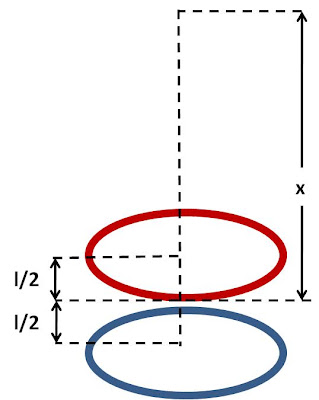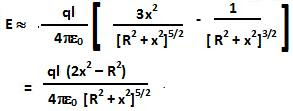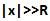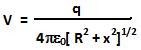## Sunday, June 6, 2010

### Irodov Problem 3.43From Problem 3.9 Eqn 4, we already know that the field due to a ring of radius R with charge q at a distance x along its axis is given by,Hence the electric field due to the two rings is given by,For, we can use Taylor's series with l as the variable around zero and approximate the electric field as,Whenthe electric field becomes,We also know that the potential due to a ring of radius R with charge q at a distance x along the axis is given by,Hence, the potential due to the two rings is given by,For, we can use Taylor's series with l as the variable around zero and approximate the potential as,